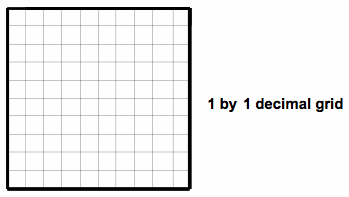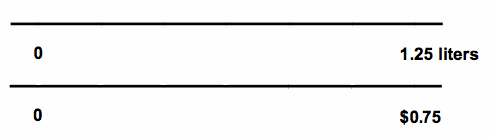# Two Models of Decimal Division

We can apply the two interpretations of division (“how many groups?” and “how many/much in one group?” for whole numbers and for fractions to decimals.

1. Use a “how many groups?” interpretation to represent 0.75 ÷ 0.25 on the grid below:2.  Think about a “how much in one group?” story problem for 0.75 (dollars) ÷ 1.25 (liters) and show your process in following double number line model.